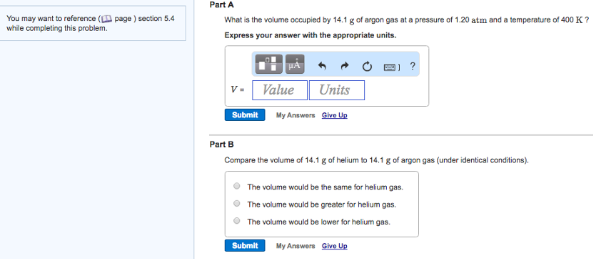# Problem: Part AWhat is the volume occupied by 14.1 g of argon gas at a pressure of 1.20 atm and a temperature of 400 K? Express your answer with the appropriate units. Part B Compare the volume of 14.1 g of helium to 14.1 g of argon gas (under identical conditions).                       • The volume would be the same for helium gas.                          • The volume would be greater for helium gas.                        • The volume would be lower for helium gas.

###### FREE Expert Solution###### Problem Details

Part A

What is the volume occupied by 14.1 g of argon gas at a pressure of 1.20 atm and a temperature of 400 K?

Part B

Compare the volume of 14.1 g of helium to 14.1 g of argon gas (under identical conditions).

• The volume would be the same for helium gas.

• The volume would be greater for helium gas.

• The volume would be lower for helium gas.